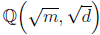# Fibonacci primes of special forms

Diana Savin
Notes on Number Theory and Discrete Mathematics, ISSN 1310-5132
Volume 20, 2014, Number 2, Pages 10—19

## Details

### Authors and affiliations

Diana SavinOvidius University of Constanta
Faculty of Mathematics and Computers Science
Bd. Mamaia no. 124, Constanta, 900527, Romania

### Abstract

A study of Fibonacci primes of the form x2 + ry2 (where r = 1; r = prime or r = perfect power) is provided.

### Keywords

• Fibonacci numbers
• Computational number theory
• Algebraic number theory computations

• 11D25
• 11S15
• 11Y40
• 11Y50

### References

1. Azizi, A., A. Mouhib, Sur le rang du 2-groupe de classes deou m = 2 ou un premier p ≡ 1 (mod 4). Transactions of the American Mathematical Society, Vol. 353, 2001, No. 7, 2741–2752.
2. Ballot, C., F. Luca, On the equation x2 + dy2 = Fn, Acta Arithmetica, Vol. 172, 2007, No. 2, 145–155.
3. Cox, D. Primes of the Form x2 + ny2: Fermat, Class Field Theory, and Complex Multiplication, A Wiley – Interscience Publication, New York, 1989.
4. Kubota,T. Uber den bizyklischen biquadratischen Zahlkorper. Nagoya Math. Journal, Vol. 10, 1956, 65–85.
5. Lehmer, E. On the Cubic Character of Quadratic Units. Journal of Number Theory, Vol. 5, 1973, 385–389.
6. Lehmer, E. On the quartic character of quadratic units. J. Reine Angew. Math., Vol. 268/269, 1974, 294–301.
7. Lemmermeyer, F. Reciprocity laws, from Euler to Eisenstein. Springer-Verlag, Heidelberg, 2000.
8. Mouhib, A. On the parity of the class number of multiquadratic number fields. Journal of Number Theory, Vol. 129, 2009, 1205–1211.
9. Savin, D., M. Stefanescu, Lectures of Arithmetic and Number theory, Edit. Matrix Rom, 2008 (in Romanian).
10. Savin, D., Stefanescu, M. A necessary condition for certain Primes to be written in the form xq + ryq, Journal of Algebra and Its Applications, Vol. 10, 2011, No. 3, 435–443.
11. Sun, Z. H. Congruences for Fibonacci numbers, http://www.hytc.cn/xsjl/szh
12. Sun, Z. H., Z.W. Sun. Fibonacci numbers and Fermat’s last theorem. Acta Arithemtica, Vol. 60, 1992, 371–388.
13. Sun, Z. H. Constructing x2 for primes p = ax2 + by2, Adv. in Appl. Math., Vol. 48, 2012, No. 1, 106–120.
14. http://www.mcs.surrey.ac.uk/Personal/R.Knott/Fibonacci/fibmaths.html
15. Magma algebra system, http://magma.maths.usyd.edu.au/magma/

## Cite this paper

APA

Savin, D. (2014). Fibonacci primes of special forms. Notes on Number Theory and Discrete Mathematics, 20(2), 10-19.

Chicago

Savin, Diana. “Fibonacci Primes of Special Forms.” Notes on Number Theory and Discrete Mathematics 20, no. 2 (2014): 10-19.

MLA

Savin, Diana. “Fibonacci Primes of Special Forms.” Notes on Number Theory and Discrete Mathematics 20.2 (2014): 10-19. Print.